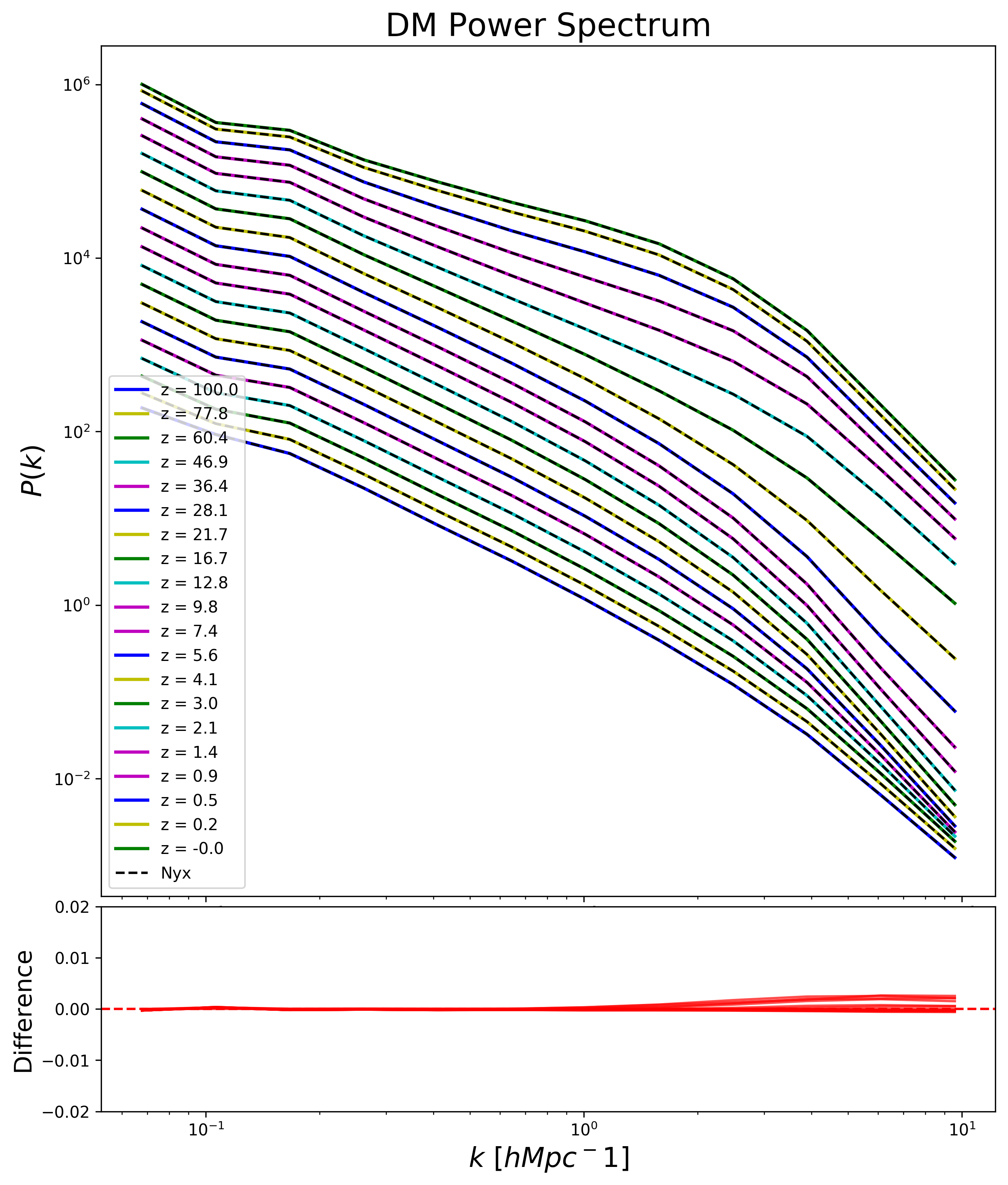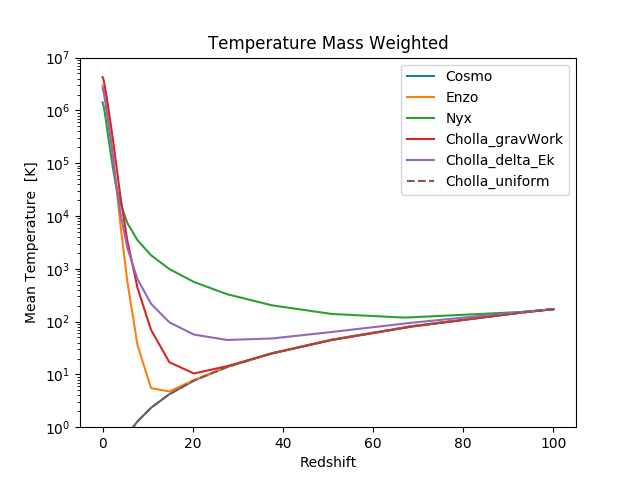### Updated DM-ONLY power spectrum:

The gravitational constant was different from the one in Nyx at the 5th digit, here are the new results, max differences are 0.25%### Gas Temperature:

Cholla gravWork : The energy update is $\Delta E = dt \rho( v \cdot g)$

Cholla deltaEk : The energy update is equal to the change in kinetic energy after the gravity update on the momentum.

Cholla uniform : Evolution of a uniform gas under adiabatic cosmological expansion, it follows the $(1+z)^2$ scaling expected for a $\gamma = 5/3$ gas.### Density - Temperature Slices:

Panel 1: Evolution with Nyx.

Panel 2: Evolution with Cholla using same initial conditions as Nyx using the change in kinetic energy for the energy update.

Panel 3: Evolution with Cholla using same initial conditions as Nyx using $\Delta E = dt \rho( v \cdot g)$ for the energy update.

Panel 4: Evolution with Enzo.

Panel 5: Evolution with Cholla using same initial conditions as Enzo using $\Delta E = dt \rho( v \cdot g)$ for the energy update.